# Can we predict stock prices with Grails Finance?

This entry is part of 15 in the series Grails Finance

Grails Finance 0.8

Yes, we can. Not always correctly, of course. One way to predict things, is to try to find the probability distribution for a phenomenon. Usually a distribution is charted as a histogram, so that is what I did. I have a lot of ground to cover today, so I will be skipping some details here and there.

## Services

I went a bit overboard with all kinds of Grails services. These are the services I made

• HistoricalQueryService – retrieves historical data from the database.
• FrequenceService calculates frequencies.
• SubtractService – subtracts vectors and calculates deltas (current – previous day).
• LnService – calculates ln var and ln var – ln var previous day.

### Historical query service

The job of the historical query service is to get from the database historical data based on a symbol or symbols and a field name. For instance, DIA and Close. When two symbols are specified the service matches the data of the two instruments based on the added timestamp and subtracts the data. Here is the code

```1 2 3 4 5 6 7 8 9 10 11 12 13 14 15 16 17 18 19 20 21 22 23 24 25 26 27 28 29 30 31 32 33 34 35 36 ... def queryVals(symbol,fieldName) { def instrument = Instrument.findBySymbol(symbol) def field = Field.findByName(fieldName) def fieldVals = FieldValue.findAllByInstrumentAndField(instrument, field) def data = []   fieldVals.each { data << Double.valueOf( it.val )}   return data }   def queryPairVals(symbol, symbol2, fieldName) { def instrument = Instrument.findBySymbol(symbol) def instrument2 = Instrument.findBySymbol(symbol2) def field = Field.findByName(fieldName) def fieldVals = FieldValue.findAllByInstrumentAndField(instrument, field) def fieldVals2 = FieldValue.findAllByInstrumentAndField(instrument2, field)   def data = []   for(int i = 0; i < fieldVals.size() && i < fieldVals2.size(); i++) { def sameDateVal = fieldVals2.each { if(fieldVals[i].added == it.added) { return it.val } }   if(sameDateVal == null) continue   data << Double.valueOf(fieldVals[i].val) - Double.valueOf(sameDateVal.val) }   return data } ...```

and here is the test code

```1 2 3 4 5 6 7 8 9 10 11 12 13 14 15 16 17 18 19 20 21 22 23 24 25 26 27 28 29 30 31 32 33 34 35 36 37 38 39 40 41 42 43 44 45 46 47 48 49 50 51 52 53 54 55 56 ... void testQueryVals() { def type = new InstrumentType(type:"type", description:"desc") assertNotNull "Type should not be null", type.save()   def ds = new Datasource(name:"DASOURCE", description: "desc") assertNotNull "DS should not be null", ds.save()   def ins = new Instrument(name: "TEST", symbol: "TEST", source: ds, instrumentType : type)   assertNotNull "Instrument should not be null",ins.save()   def f = new Field(name:"TOAST", description: "desc") assertNotNull "f should not be null", f.save()   def v = new FieldValue(instrument:ins, field:f, added: new DateTime(), val: "1.0") assertNotNull "V should not be null", v.save()   def vals = historicalQueryService.queryVals("TEST", "TOAST") assertNotNull "Vals should not be null",vals assertEquals "There should be 1 value", vals.size() , 1 }   void testQueryPairVals() { def type = new InstrumentType(type:"type", description:"desc") assertNotNull "Type should not be null", type.save()   def ds = new Datasource(name:"DASOURCE", description: "desc") assertNotNull "DS should not be null", ds.save()   def ins = new Instrument(name: "TEST", symbol: "TEST", source: ds, instrumentType : type) def ins2 = new Instrument(name: "TEST2", symbol: "TEST2", source: ds, instrumentType : type)   assertNotNull "Instrument should not be null",ins.save() assertNotNull "Instrument should not be null",ins2.save()   def f = new Field(name:"TOAST", description: "desc") assertNotNull "f should not be null", f.save()   def now = new DateTime() def v = new FieldValue(instrument:ins, field:f, added: now, val: "1.0") def v2 = new FieldValue(instrument:ins2, field:f, added: now, val: "3.0") assertNotNull "V should not be null", v.save() assertNotNull "V2 should not be null", v2.save()   def vals = historicalQueryService.queryPairVals("TEST", "TEST2", "TOAST") assertNotNull "Vals should not be null",vals assertEquals "There should be 1 value", vals.size() , 1 } ...```

### Frequence service

The frequence service defines a number of bins and counts the number of occurrences in each bin. I defined a Bin class as follows

```1 2 3 4 5 6 7 8 9 10 11 12 13 ... double start double end double mid String label   Bin(s, e) { start = s end = e mid = (start + end) / 2 label = sprintf('%.3g', mid) } ...```

The service code is shown below. First I calculate the size of bin, then I make a list of Bin objects. After that I check in which bin each input value falls and increase the count for the lucky bin. There is also a convenience method that reduces the x labels by a factor of million – this is handy for volumes.

```1 2 3 4 5 6 7 8 9 10 11 12 13 14 15 16 17 18 19 20 21 22 23 24 25 26 27 28 29 30 31 32 33 34 35 36 37 38 39 40 41 42 43 44 45 46 47 48 49 50 51 52 53 54 55 ... def frequence(input) { def step = calcStep(input) def bins = makeBins(input.min(), step) def frequency = [:]   for( int i in 0..<NBINS) { frequency[ bins[i].label ] = 0 }   def size = input.size()   input.each { def index = calcIndex(it, bins) frequency[ bins[index].label]++ }   return frequency }   def makeBins(min, step) { def bins = []   for( int i in 0..<NBINS) { bins << new Bin(min + i * step, min + (i + 1) * step) }   return bins }   def reduceKeys(frequency) { def reduced = [:]   frequency.each { key,value -> reduced[Math.round(Double.valueOf(key)/(1000 * 1000))] = value }   return reduced }   def calcIndex(it, bins) { for(int i in 0..<bins.size()) { if(it >= bins[i].start && it < bins[i].end) { return i } }   return bins.size() - 1 }   def calcStep(input) { return (input.max() - input.min())/ NBINS } ...```

Here is some of the test code

```1 2 3 4 5 6 7 8 9 10 11 12 13 14 15 16 17 18 ... void testFrequence() { assertNotNull frequenceService def frequencies = frequenceService.frequence([1.0, 1.5, 32.0]) assertNotNull frequencies assertEquals "Number of bins incorrect", 30, frequencies.size()   def store = 0   frequencies.each { key,value -> store += value }   assertEquals 3, store }   void testStep() { assertEquals 1.0, frequenceService.calcStep([0,30.0]) } ...```

### Subtract service

The subtract service subtracts vectors and calculates deltas (current – previous day).

```1 2 3 4 5 6 7 8 9 10 11 12 13 14 15 16 17 18 19 20 21 22 23 24 25 26 27 28 29 ... def minPrev(array) { def subtracted = []   for(int i in 1..<array.size() ) { subtracted << array[i] - array[i - 1] }   return subtracted }   def min(left, right) { def subtracted = []   for(int i in 0..<array.size() ) { subtracted << array[i] - array[i - 1] }   return subtracted } ... def testMinPrevious() { def subtracted = subtractService.minPrev([1, 2, 3]) assertNotNull subtracted assertEquals 2, subtracted.size() assertEquals 1, subtracted assertEquals 1, subtracted } ...```

### Ln service

The Ln service calculates the natural logarithm of a variable vector and the difference of the ln of a variable and the ln of the previous variable value. There is a small problem with logarithms. You cannot take the logarithm of 0 or negative numbers. So I cheat a little by substituting negative numbers by their absolute value and avoiding 0.

```1 2 3 4 5 6 7 8 9 10 11 12 13 14 15 16 17 18 19 20 21 22 23 ... def ln(numbers) { def result = []   numbers.each { if(it != 0) result << Math.log(Math.abs(it)) }   return result }   def deltaLn(numbers) { def result = []   for(int i in 1..<numbers.size()) { if(numbers[i] != 0 && numbers[i - 1] != 0) result << Math.log(Math.abs(numbers[i])) - Math.log(Math.abs(numbers[i-1])) }   return result } ...```

Some more tests

```1 2 3 4 5 6 7 8 9 10 11 12 13 14 15 ... void testLn() { def outcome = lnService.ln([-1,0, 1]) assertNotNull outcome assertEquals 2, outcome.size() assertEquals 0, outcome }   void testDeltaLn() { def outcome = lnService.deltaLn([-1,0,1,1]) assertNotNull outcome assertEquals 1, outcome.size() assertEquals 0, outcome } ...```

## Controller

Services on their own don’t do much, so we need a controller to use the services and communicate with the views.

```1 2 3 4 5 6 7 8 9 10 11 12 13 14 15 16 17 18 19 20 21 22 23 24 25 26 27 28 29 30 31 32 33 34 35 36 37 38 39 40 41 42 43 44 45 46 47 48 49 50 51 52 53 54 55 56 ... def calcFrequence(vals, fieldVar) { def frequence = frequenceService.frequence(vals)   if(params.fieldVar == 'Volume') { frequence = frequenceService.reduceKeys(frequence) }   return frequence }   def calcDeltaFrequence(vals) { def subtracted = subtractService.minPrev(vals) return frequenceService.frequence(subtracted) }   def calcLnFrequency(vals) { def lned = lnService.ln(vals) return frequenceService.frequence(lned) }   def calcDeltaLnFrequency(vals) { def deltaLned = lnService.deltaLn(vals) return frequenceService.frequence(deltaLned) }   def makeResponse(vals) { def frequence = calcFrequence(vals, params.fieldVar)   def deltasFrequency = calcDeltaFrequence(vals)   def lnFrequency = calcLnFrequency(vals)   def deltaLnFrequency = calcDeltaLnFrequency(vals)   return [data : frequence, deltaData : deltasFrequency, lnData : lnFrequency, deltaLnData : deltaLnFrequency] }   def index = { if(params.fieldVar != null) { def vals = historicalQueryService.queryVals( params.select1, params.fieldVar); return makeResponse(vals) } }   def pairs = { if(params.fieldVar != null) { def vals = historicalQueryService.queryPairVals( params.select1, params.select2, params.fieldVar); return makeResponse(vals) } } ...```

## Views

There are 2 views – the main view for a single symbol and a view for a pair of symbols.

### Main view

The view consists of a form with 4 histograms beneath it. The form lets you choose a symbol and a field. The histograms are made with the Google Charts Grails plugin.

```1 2 3 4 5 6 7 8 9 10 11 12 13 14 15 16 17 18 19 20 21 22 23 24 25 26 27 28 29 30 31 32 33 34 35 36 37 38 39 40 41 42 43 44 45 46 47 48 49 50 51 52 53 54 55 56 57 58 59 60 61 62 63 64 65 66 67 68 69 70 71 72 73 74 75 76 77 78 79 80 81 82 83 84 85 86 87 88 89 90 91 92 93 94 95 96 <html> <head> <title>Historical data histogram</title> </head> <body> <table> <tr> <g:form> <td> <g:select name="select1" from="\${['DIA', 'SPY', 'GLD']}" value="\${params.select1}"/> </td> <td> <g:select name="fieldVar" from="\${['Open', 'High', 'Low', 'Close', 'Volume']}" value="\${params.fieldVar}"/> </td> <td> <g:actionSubmit name="doChart" action="index" value="Chart"/> </td> </g:form></td> </tr> </table>   <g:if test="\${data != null}"> <g:barChart type="bvs" title="Histogram \${params.select1} (\${params.fieldVar})" size="\${[900,200]}" colors="\${['00FF00']}" fill="\${'bg,s,efefef' }" axes="x,y" axesLabels="\${ [0:data.keySet(), 1:[0,data.values().max()/2, data.values().max()] ] }" dataType="simple" data="\${data.values().asList()}" /> </g:if>   <br/> <br/>   <g:if test="\${deltaData != null}"> <g:barChart type="bvs" title="Histogram \${params.select1} (\${params.fieldVar} - previous day \${params.fieldVar}) " size="\${[900,200]}" colors="\${['00FF00']}" fill="\${'bg,s,efefef' }" axes="x,y" axesLabels="\${ [0:deltaData.keySet(), 1:[0,deltaData.values().max()/2, deltaData.values().max()] ] }" dataType="simple" data="\${deltaData.values().asList()}" /> </g:if>   <br/> <br/>   <g:if test="\${lnData != null}"> <g:barChart type="bvs" title="Histogram \${params.select1} (Log \${params.fieldVar}) " size="\${[900,200]}" colors="\${['00FF00']}" fill="\${'bg,s,efefef' }" axes="x,y" axesLabels="\${ [0:lnData.keySet(), 1:[0,lnData.values().max()/2, lnData.values().max()] ] }" dataType="simple" data="\${lnData.values().asList()}" /> </g:if>   <br/><br/>   <g:if test="\${deltaLnData != null}"> <g:barChart type="bvs" title="Histogram \${params.select1} (Log \${params.fieldVar} - Log previous day \${params.fieldVar}) " size="\${[900,200]}" colors="\${['00FF00']}" fill="\${'bg,s,efefef' }" axes="x,y" axesLabels="\${ [0:deltaLnData.keySet(), 1:[0,deltaLnData.values().max()/2, deltaLnData.values().max()] ] }" dataType="simple" data="\${deltaLnData.values().asList()}" /> </g:if> </body> </html>```

### The pairs view

The pairs view is almost a copy of the main view. The difference being that in this view you can specify an extra symbol.

## Result

The result is displayed in the table below for GLD, SPY, DIA and their respective differences. The data comes from historical data of end of day OHLC prices and volume.

 Open High Low Close Volume DIA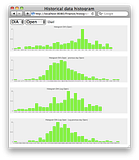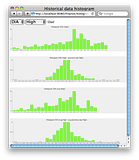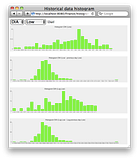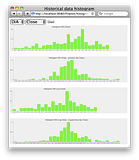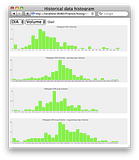SPY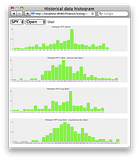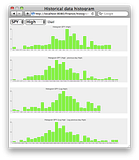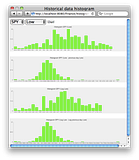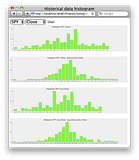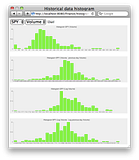GLD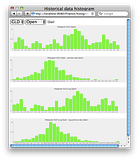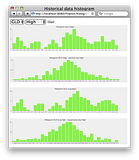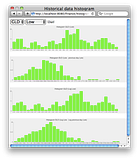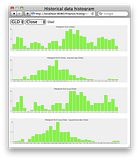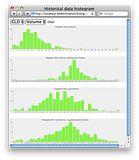DIA – SPY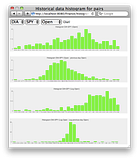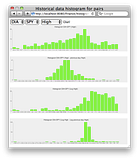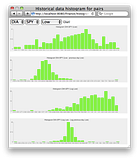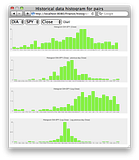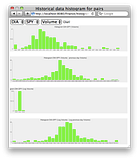DIA – GLD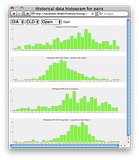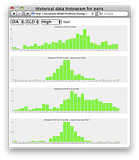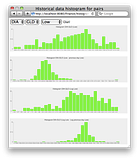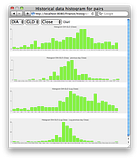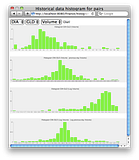SPY – GLD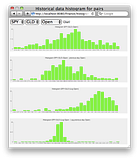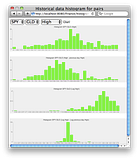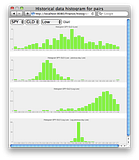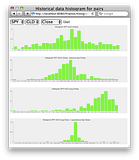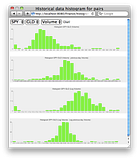## Conclusions

Turns out that the distributions are pretty non random, almost Gaussian. So we may be able to predict stock prices, a little a bit more accurately than you would have expected.

By the author of NumPy Beginner's Guide, NumPy Cookbook and Instant Pygame. If you enjoyed this post, please consider leaving a comment or subscribing to the RSS feed to have future articles delivered to your feed reader.
This entry was posted in programming and tagged , , . Bookmark the permalink.

### 3 Responses to Can we predict stock prices with Grails Finance?

1.Peter says:

Hi there,

I am a novice grails developer and just managed to run your app at http://grails-finance.googlecode.com/svn/trunk/

Is there any chance that you might commit the latest version of grails-finance or have some seed script to populate the database?

Just trying to understand what your blog, and your app, are saying

Have a great daY!

Regards,
Peter

2.admin says:

Thank you Peter,

Unfortunately I don’t have a seed script for the database. It is a very good idea and I will try to find some time to work on it, but I cannot promise anything.

Regards,

Ivan